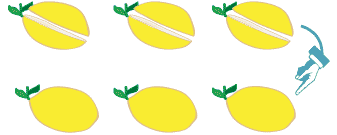### Multiply and divide decimals

A strong understanding of the multiplication and division algorithm used with whole numbers and a clear knowledge of place value are important. When decimal numbers are introduced, you will be able to extend your understanding of the multiplication and division algorithms to incorporate the new information.

#### Multiply decimals by whole numbers

To help you understand how to multiply decimals, it can be useful to use objects to demonstrate what is happening.

For a multiplication, such as 0.5 × 6, modelling the situation with objects can help.

From working with fractions, we know that 0.5 is the same as one half. We can use lemons to show six lots of one half:Detailed description

Six lots of $$\dfrac{1}{2}$$ a lemon = three whole lemons.
6 × $$\dfrac{1}{2}$$ = 3
Six lots of 0.5 a lemon = three whole lemons.
6 × 0.5 = 3
This demonstrates that even though we are multiplying by 6, the result is actually smaller than 6.

For the multiplication 0.5 × 6 we might convert to fractions:

\begin{align*} 0.5 \times 6 &= \frac{1}{2} \times 6 \\ &= \frac{1}{2} \times \frac{6}{1} \\ &= \frac{6}{2} \\ &= 3 \end{align*}

\begin{align*} 0.5 \times 6 &= 0.5 + 0.5 + 0.5 + 0.5 + 0.5 + 0.5 \\ &= 3 \end{align*}

We can do a simple multiplication:

0.5 × 6 = 3.0

In our head we think 5 × 6 = 30, but the solution must have the same number of decimal places as the numbers we are multiplying. Thinking of 3.0 instead of 30 helps with this.

Or the equation could be set out using the multiplication algorithm we used for whole numbers:

 0 . 5 × 6 3 . 0

Note that there is a total of one decimal place in the two numbers being multiplied and one decimal place in the solution.

Let’s look at another example: If we buy four 2.75 litre bottles of juice, how much juice is there?

0.75 of a litre is the same as $$\dfrac{3}{4}$$ of a litre. So if we convert to fractions:

\begin{align*}2.75 \times 4 &= 2 \frac{3}{4} \times 4 \\ &= \frac{11}{4}  \times \frac{4}{1} \\ &= 11\end{align*}

Using repeated addition is shown here.

\begin{align*}2.75\times 4 &= 2\frac{3}{4} +2\frac{3}{4} +2\frac{3}{4} +2\frac{3}{4} \\ &= 11\end{align*}

We can use the multiplication algorithm.

 $$^3 2$$ . $$^2 7$$ 5 × 4 1 1 . 0 0

Note that there is a total of two decimal places in the two numbers being multiplied and two decimal places in the solution.